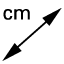# Equilateral and Equiangular Triangle Theorems

## Question 1:

Measure the angles on the Equilateral Triangle using the angle measurement tool. Are the angles congruent?

Select all that apply
• A
• B

## Question 2:

What is the measure of each angle in the Equilateral Triangle?

## Question 3:

Measure the sides on the Equiangular Triangle using the distance measurement tool. Are the sides congruent?

Select all that apply
• A
• B